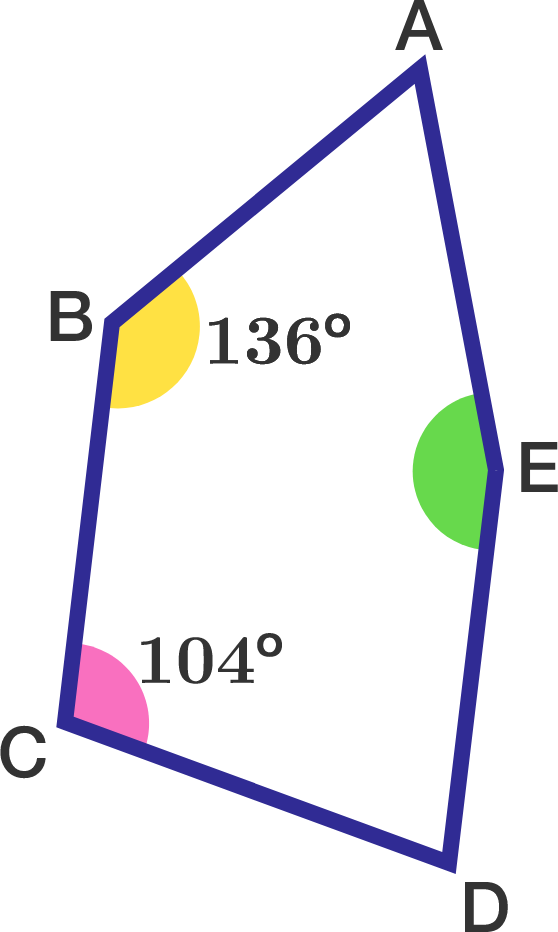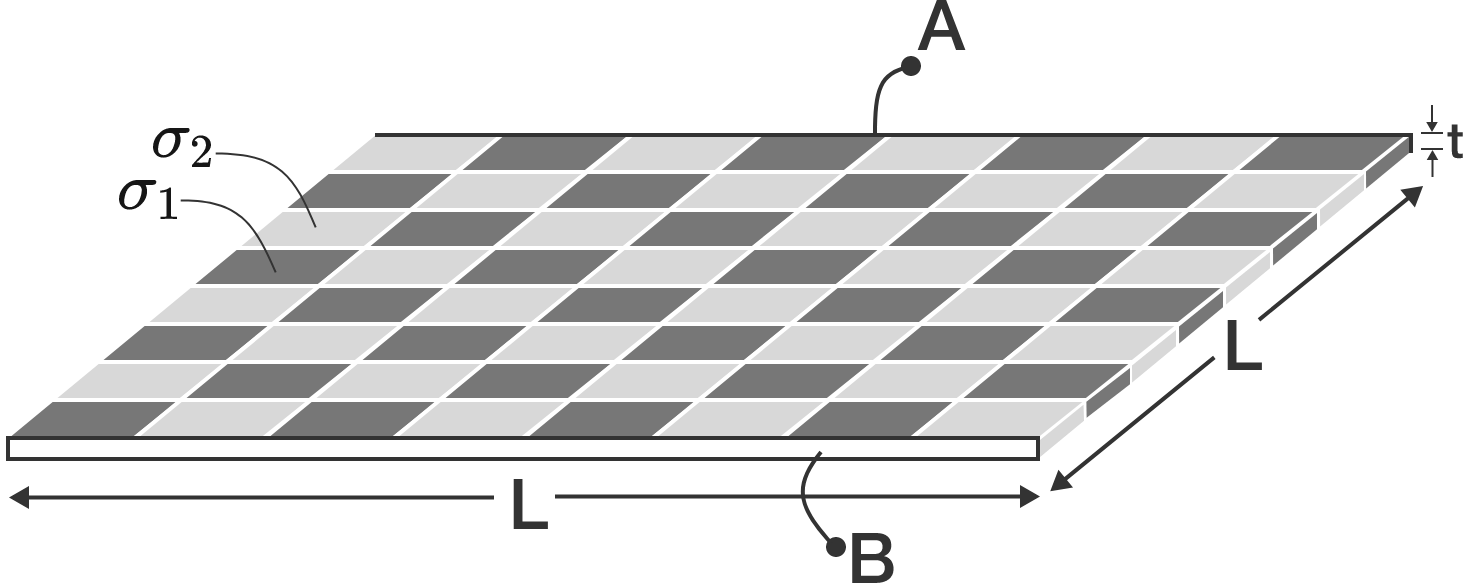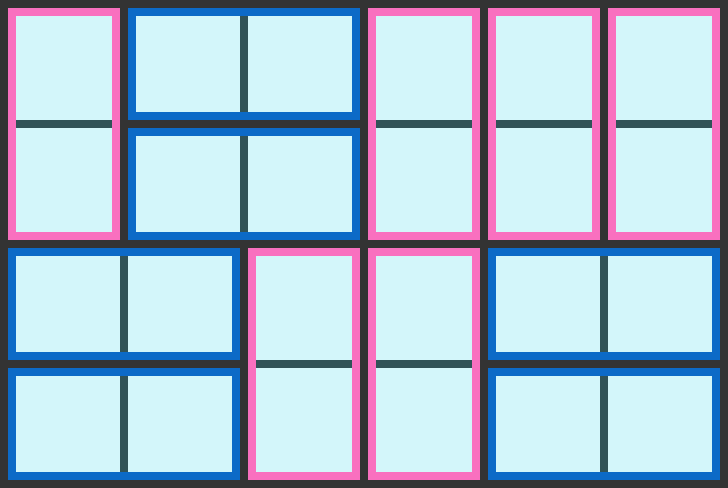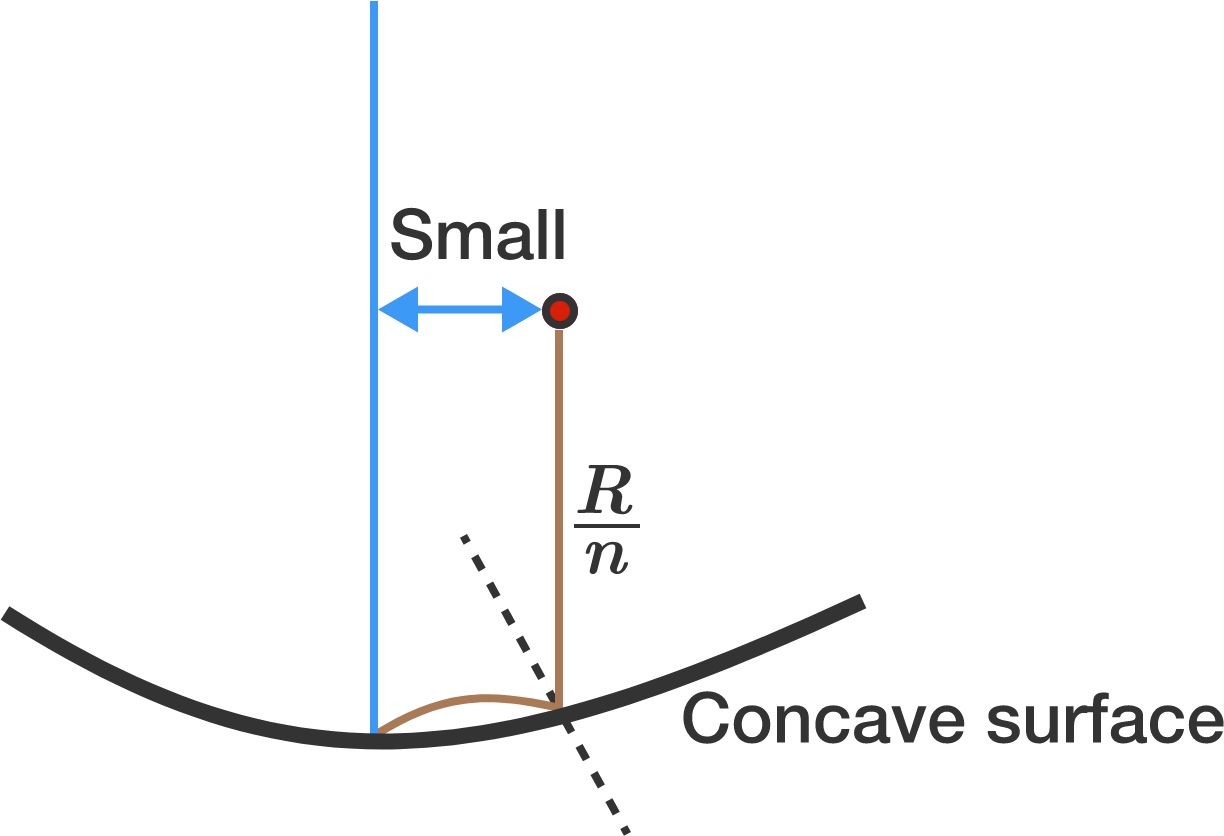# Problems of the Week

Contribute a problem

# 2017-03-20 Advanced

Does there exist a function $f: \mathbb{R} \rightarrow \mathbb{R}$ that is continuous on exactly the rational numbers?

Note: The following function $f: \mathbb{R} \rightarrow \mathbb{R}$ is discontinuous on exactly the rational numbers:

$\hspace{1.0cm}$ Index all the rational numbers using the bijection function $a: \mathbb{N} \rightarrow \mathbb{Q}$.
$\hspace{1.0cm}$ Define $f: \mathbb{R} \rightarrow \mathbb{R}$ as $\displaystyle f(x) = \sum_{ a(n) < x } 2^{-n }.$

Let $ABCDE$ be an equilateral convex pentagon such that $\angle ABC=136^\circ$ and $\angle BCD=104^\circ$. What is the measure (in degrees) of $\angle AED$?Note: An equilateral polygon is a polygon whose sides are all of the same length. It does not imply that all the internal angles are equal, nor that the polygon is cyclic.A parallel plate capacitor, with plates $\textbf{A}$ and $\textbf{B}$ of equal dimensions $t \times L$ at a distance of $L$, is filled with square tiles of dielectric to make a chess-board-like capacitor, as shown in the picture above.

Dielectric constant of the dark tile is $\sigma_1,$ that of the light tile is $\sigma_2,$ and $\epsilon_0$ is the permittivity of free space. All the dielectric tiles are square cuboids of thickness $t$.

Find the capacitance of this capacitor.


Details and Assumptions:

• Assume that the electric field varies between the plates like an ideal parallel plate capacitor.

How many ways are there to tile a $4\times6$ rectangle with twelve $1\times2$ dominoes?A ball with zero initial velocity falls from a height of $\frac{R}{n}$ near the vertical axis of symmetry on a concave spherical surface of radius $R$. Assuming that the collision is elastic, it is observed that the second impact of the ball is at the lowest point of the spherical surface. Determine the value of $n$ to the nearest integer.

×

Problem Loading...

Note Loading...

Set Loading...